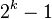# Hamming code facts for kids

Kids Encyclopedia Facts

A hamming code is an error-correcting block code. The code is named after Richard Hamming who developed it in the 1950s. At the time, Hamming worked with machines that had relays and used punched cards to read the data. Because they were heavily used, the punched cards often had errors, which needed to be corrected by employees.

Hamming codes are used for digital signal processing and telecommunications. Hamming codes are generated according to certain rules. Hamming codes use multiple parity bits. A parity bit tells whether a group of bits is even or odd. In a hamming code, each bit of data is covered by several parity bits. This allows to detect errors, and in certain cases, to correct them as well. A hamming code uses redundancy. If there are three parity bits per code word, the code word must have a length of 7 ($2^k - 1$, for k as the number of parity bits). This leaves 4 bits of user data per code word, in the example. Usually, this is written as (N,n), where the first number is the total length of a code word, and the second is the number of bits for user data. The example above is (7,4).

The shortest possible Hamming code is (3,1), 2 parity bits are used for one data bit. This code has two valid values 000 and 111 - The codes 001, 010 and 100 are transmission errors, and will be assigned to the valid code word 000. The other possibilities 011,101 and 110 will be changed to '111.Hamming code Facts for Kids. Kiddle Encyclopedia.